# Stokes Theorem

Stokes Theorem (also known as Generalized Stoke’s Theorem) is a declaration about the integration of differential forms on manifolds, which both generalizes and simplifies several theorems from vector calculus. As per this theorem, a line integral is related to a surface integral of vector fields. Learn the stokes law here in detail with formula and proof.

## Stokes’ Theorem Formula

The Stoke’s theorem states that “the surface integral of the curl of a function over a surface bounded by a closed surface is equal to the line integral of the particular vector function around that surface.”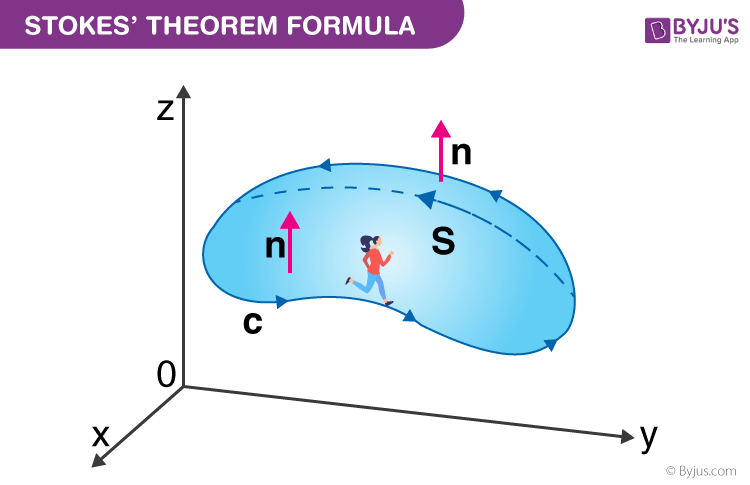$$\begin{array}{l}\oint _{C} \vec{F}.\vec{dr} = \iint_{S}(\bigtriangledown \times \vec{F}). \vec{dS}\end{array}$$

Where,

C = A closed curve.

S = Any surface bounded by C.

F = A vector field whose components have continuous derivatives in an open region of R3 containing S.

This classical declaration, along with the classical divergence theorem, fundamental theorem of calculus, and Green’s theorem are exceptional cases of the general formulation specified above.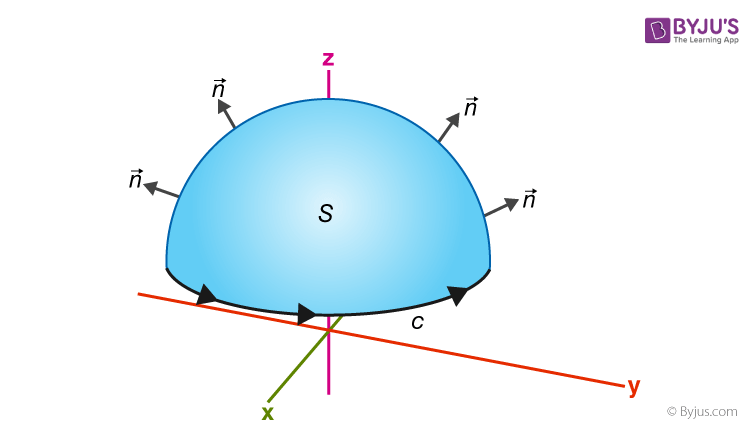This means that:

If you walk in the positive direction around C with your head pointing in the direction of n, the surface will always be on your left.

S is an oriented smooth surface bounded by a simple, closed smooth-boundary curve C with positive orientation.

### Stokes Theorem Statement

The line integral around S (the boundary curve) of F’s tangential component is equal to the surface integral of the normal component of the curl of F.

The positively oriented boundary curve of the oriented surface S will be ∂S.

Thus, stokes theorem can also be expressed as:

$$\begin{array}{l}\int\int_{S}curl\vec{F}.d\vec{S} = \int_{\partial S}\vec{F}.d\vec{r}\end{array}$$

## Gauss Divergence theorem

The Gauss divergence theorem states that the vector’s outward flux through a closed surface is equal to the volume integral of the divergence over the area within the surface. Put differently, the sum of all sources subtracted by the sum of every sink results in the net flow of an area.

Gauss divergence theorem is a result that describes the flow of a vector field by a surface to the behaviour of the vector field within the surface.

## Stokes’ Theorem Proof

We assume that the equation of S is Z = g(x, y), (x, y)D

Where g has a continuous second-order partial derivative.

D is a simple plain region whose boundary curve C1 corresponds to C.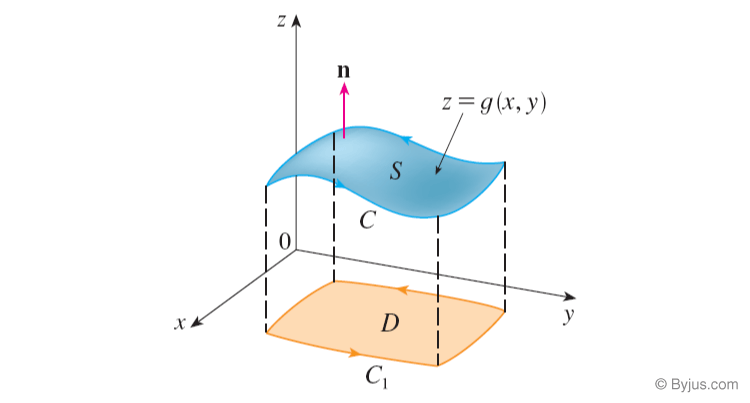This can be explained easily with 3D air projection at BYJU’S – The learning app where all concepts like the same are explained in great detail.

## Stokes Theorem Example

Example:

Using stokes theorem, evaluate:

$$\begin{array}{l}\int\int_{S}curl\vec{F}.d\vec{S},\ where\vec{F}= xz\hat{i} + yz\hat{j} + xy\hat{k},\end{array}$$
such that S is the part of the sphere x2 + y2 + z2 = 4 that lies inside the cylinder x2 + y2 = 1 and above the xy-plane.

Solution:

Given,

Equation of sphere: x2 + y2 + z2 = 4….(i)

Equation of cylinder: x2 + y2 = 1….(ii)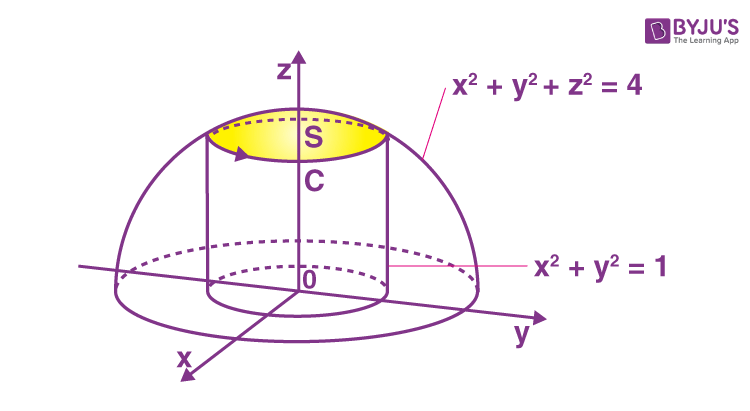Subtracting (ii) from (i),

z2 = 3

z = √3 (since z is positive)

Now,

The circle C is will be: x2 + y2 = 1, z = √3

The vector form of C is given by:

$$\begin{array}{l}r(t)= cos t\hat{i} + sin t\hat{j} + \sqrt{3}\hat{k}; 0\le t\le 2\pi\end{array}$$

$$\begin{array}{l}Thus,\ r'(t)= -sin t\hat{i} + cos t\hat{j}\end{array}$$

Let us write F(r(t)) as:

$$\begin{array}{l}F(r(t))=\sqrt{3}cos t\ \hat{i}+\sqrt{3}sin t\ \hat{j}+cost\ sin t \ \hat{k}\end{array}$$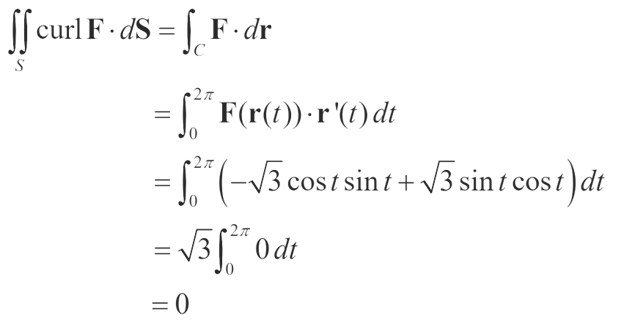### Stokes Theorem Applications

Stokes’ theorem provides a relationship between line integrals and surface integrals. Based on our convenience, one can compute one integral in terms of the other. Stokes’ theorem is also used in evaluating the curl of a vector field.  Stokes’ theorem and the generalized form of this theorem are fundamental in determining the line integral of some particular curve and evaluating a bounded surface’s curl. Generally, this theorem is used in physics, particularly in electromagnetism.

### Stokes Theorem Problems

Find the below practice problems in Stokes theorem.

1. $$\begin{array}{l}\text{Use Stokes’ Theorem to evaluate} \int\int_{S} (curl \vec{F}).\vec{n} dS\ \text{when}\ \vec{F} = z^2\hat{i} + y^2\hat{j} + xy\hat{k}\ \text{and C is the triangle defined by (1, 0, 0), (0, 1, 0) and (0, 0, 2)}\end{array}$$
2. $$\begin{array}{l}\text{Verify the Stokes theorem for the vector field}\ \vec{F} = y\hat{i}+ 2z\hat{j}+x^{2} \hat{k}\ \text{and Surface S, where S is the parabola}\ z = 4 – x^{2} – y^{2}.\end{array}$$
3. $$\begin{array}{l}\text{Compute} \oint_{C}x^{2}zdx + 3xdy – y^{3}dz, \text{Where C is the unit circle}\ x^{2}+y^{2}= 1 \ \text{oriented counter-clockwise}\end{array}$$

For Stokes’ theorem examples and Stokes’ theorem problems stay tuned with BYJU’S.